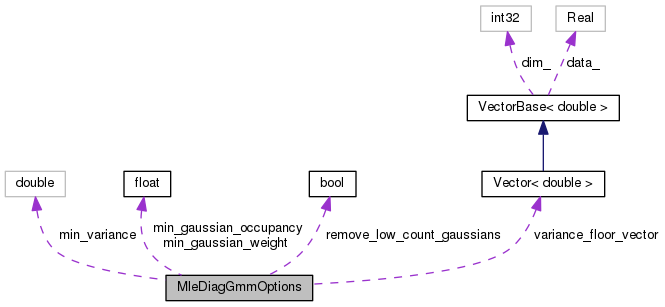MleDiagGmmOptions Struct Reference

Configuration variables like variance floor, minimum occupancy, etc. More...

`#include <mle-diag-gmm.h>`

Collaboration diagram for MleDiagGmmOptions:[legend]

## Public Member Functions

MleDiagGmmOptions ()

void Register (OptionsItf *opts)

## Public Attributes

Vector< double > variance_floor_vector
Variance floor for each dimension [empty if not supplied]. More...

BaseFloat min_gaussian_weight
Minimum weight below which a Gaussian is not updated (and is removed, if remove_low_count_gaussians == true);. More...

BaseFloat min_gaussian_occupancy
Minimum count below which a Gaussian is not updated (and is removed, if remove_low_count_gaussians == true). More...

double min_variance
Minimum allowed variance in any dimension (if no variance floor) It is in double since the variance is computed in double precision. More...

bool remove_low_count_gaussians

## Detailed Description

Configuration variables like variance floor, minimum occupancy, etc.

needed in the estimation process.

Definition at line 38 of file mle-diag-gmm.h.

## ◆ MleDiagGmmOptions()

 MleDiagGmmOptions ( )
inline

Definition at line 52 of file mle-diag-gmm.h.

52  {
53  // don't set var floor vector by default.
54  min_gaussian_weight = 1.0e-05;
56  min_variance = 0.001;
58  }
BaseFloat min_gaussian_occupancy
Minimum count below which a Gaussian is not updated (and is removed, if remove_low_count_gaussians ==...
Definition: mle-diag-gmm.h:47
double min_variance
Minimum allowed variance in any dimension (if no variance floor) It is in double since the variance i...
Definition: mle-diag-gmm.h:50
BaseFloat min_gaussian_weight
Minimum weight below which a Gaussian is not updated (and is removed, if remove_low_count_gaussians =...
Definition: mle-diag-gmm.h:44

## ◆ Register()

 void Register ( OptionsItf * opts )
inline

Definition at line 59 of file mle-diag-gmm.h.

References OptionsItf::Register().

Referenced by main().

59  {
60  std::string module = "MleDiagGmmOptions: ";
61  opts->Register("min-gaussian-weight", &min_gaussian_weight,
62  module+"Min Gaussian weight before we remove it.");
63  opts->Register("min-gaussian-occupancy", &min_gaussian_occupancy,
64  module+"Minimum occupancy to update a Gaussian.");
65  opts->Register("min-variance", &min_variance,
66  module+"Variance floor (absolute variance).");
67  opts->Register("remove-low-count-gaussians", &remove_low_count_gaussians,
68  module+"If true, remove Gaussians that fall below the floors.");
69  }
BaseFloat min_gaussian_occupancy
Minimum count below which a Gaussian is not updated (and is removed, if remove_low_count_gaussians ==...
Definition: mle-diag-gmm.h:47
double min_variance
Minimum allowed variance in any dimension (if no variance floor) It is in double since the variance i...
Definition: mle-diag-gmm.h:50
BaseFloat min_gaussian_weight
Minimum weight below which a Gaussian is not updated (and is removed, if remove_low_count_gaussians =...
Definition: mle-diag-gmm.h:44

## ◆ min_gaussian_occupancy

 BaseFloat min_gaussian_occupancy

Minimum count below which a Gaussian is not updated (and is removed, if remove_low_count_gaussians == true).

Definition at line 47 of file mle-diag-gmm.h.

Referenced by main(), kaldi::MleAmDiagGmmUpdate(), and kaldi::MleDiagGmmUpdate().

## ◆ min_gaussian_weight

 BaseFloat min_gaussian_weight

Minimum weight below which a Gaussian is not updated (and is removed, if remove_low_count_gaussians == true);.

Definition at line 44 of file mle-diag-gmm.h.

Referenced by kaldi::MleDiagGmmUpdate().

## ◆ min_variance

 double min_variance

Minimum allowed variance in any dimension (if no variance floor) It is in double since the variance is computed in double precision.

Definition at line 50 of file mle-diag-gmm.h.

Referenced by main(), kaldi::MleDiagGmmUpdate(), and UnitTestEstimateDiagGmm().

## ◆ remove_low_count_gaussians

 bool remove_low_count_gaussians

Definition at line 51 of file mle-diag-gmm.h.

Referenced by kaldi::MleAmDiagGmmUpdate(), and kaldi::MleDiagGmmUpdate().

## ◆ variance_floor_vector

 Vector variance_floor_vector

Variance floor for each dimension [empty if not supplied].

It is in double since the variance is computed in double precision.

Definition at line 41 of file mle-diag-gmm.h.

Referenced by kaldi::MleDiagGmmUpdate().

The documentation for this struct was generated from the following file: